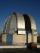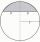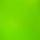# Cube and sphere

Cube with the surface area 150 cm2 is described sphere. What is sphere surface?

Result

S =  235.619 cm2

#### Solution:Leave us a comment of example and its solution (i.e. if it is still somewhat unclear...):

Showing 0 comments:Be the first to comment!#### To solve this example are needed these knowledge from mathematics:

Pythagorean theorem is the base for the right triangle calculator.

## Next similar examples:

1. Sphere areaA cube with edge 1 m long is circumscribed sphere (vertices of the cube lies on the surface of a sphere). Determine the surface area of the sphere.
2. Cube in sphereThe sphere is inscribed cube with edge 8 cm. Find the radius of the sphere.
3. Cube diagonalsCalculate the length of the side and the diagonals of the cube with a volume of 27 cm3.
4. Cube surface areaWall of cube has content area 99 cm square. What is the surface of the cube?
5. Center of the cubeCenter of the cube has distance 33 cm from each vertex. Calculate the volume V and surface area S of the cube.
6. Body diagonalFind the cube surface if its body diagonal has a size of 6 cm.
7. Wall diagonalCalculate the length of wall diagonal of the cube whose surface is 384 cm square.
8. Body diagonalCalculate the volume and surface of the cube if the body diagonal measures 10 dm.
9. Cube 8The surface of the cube is 0.54 m2. Calculate the length of the cube edge.
10. Cube edgeDetermine the edges of the cube when the surface is equal to 37.5 cm square.
11. Cube diagonalsThe cube has a wall area of 81 cm square. Calculate the length of its edge, wall, and body diagonal.
12. ObservatoryObservatory dome has the shape of a hemisphere with a diameter d = 10 m. Calculate the surface.
13. Billiard ballsA layer of ivory billiard balls of radius 6.35 cm is in the form of a square. The balls are arranged so that each ball is tangent to every one adjacent to it. In the spaces between sets of 4 adjacent balls other balls rest, equal in size to the original. T
14. Spherical capWhat is the surface area of a spherical cap, the base diameter 20 m, height 2 m.
15. The ditchDitch with cross section of an isosceles trapezoid with bases 2m 6m are deep 1.5m. How long is the slope of the ditch?
16. Two diagonalsThe rhombus has a side length 12 cm and length of one diagonal 21 cm. What is the length of the second diagonal?
17. Square2Side of the square is a = 6.2 cm, how long is its diagonal?